Скачать презентацию Unit 9 -Right Triangle Trigonometry This unit

45b8bea4f7a77fe5a56f1a5eb5b9666f.ppt

• Количество слайдов: 49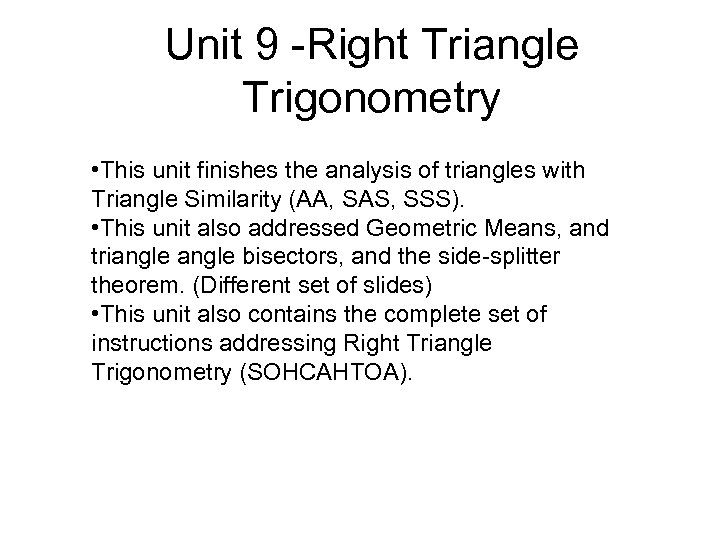Unit 9 -Right Triangle Trigonometry • This unit finishes the analysis of triangles with Triangle Similarity (AA, SAS, SSS). • This unit also addressed Geometric Means, and triangle bisectors, and the side-splitter theorem. (Different set of slides) • This unit also contains the complete set of instructions addressing Right Triangle Trigonometry (SOHCAHTOA).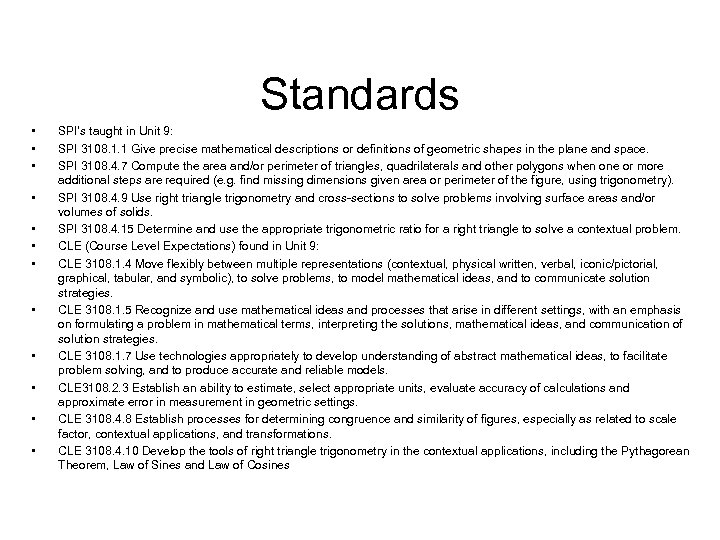Standards • • • SPI’s taught in Unit 9: SPI 3108. 1. 1 Give precise mathematical descriptions or definitions of geometric shapes in the plane and space. SPI 3108. 4. 7 Compute the area and/or perimeter of triangles, quadrilaterals and other polygons when one or more additional steps are required (e. g. find missing dimensions given area or perimeter of the figure, using trigonometry). SPI 3108. 4. 9 Use right triangle trigonometry and cross-sections to solve problems involving surface areas and/or volumes of solids. SPI 3108. 4. 15 Determine and use the appropriate trigonometric ratio for a right triangle to solve a contextual problem. CLE (Course Level Expectations) found in Unit 9: CLE 3108. 1. 4 Move flexibly between multiple representations (contextual, physical written, verbal, iconic/pictorial, graphical, tabular, and symbolic), to solve problems, to model mathematical ideas, and to communicate solution strategies. CLE 3108. 1. 5 Recognize and use mathematical ideas and processes that arise in different settings, with an emphasis on formulating a problem in mathematical terms, interpreting the solutions, mathematical ideas, and communication of solution strategies. CLE 3108. 1. 7 Use technologies appropriately to develop understanding of abstract mathematical ideas, to facilitate problem solving, and to produce accurate and reliable models. CLE 3108. 2. 3 Establish an ability to estimate, select appropriate units, evaluate accuracy of calculations and approximate error in measurement in geometric settings. CLE 3108. 4. 8 Establish processes for determining congruence and similarity of figures, especially as related to scale factor, contextual applications, and transformations. CLE 3108. 4. 10 Develop the tools of right triangle trigonometry in the contextual applications, including the Pythagorean Theorem, Law of Sines and Law of Cosines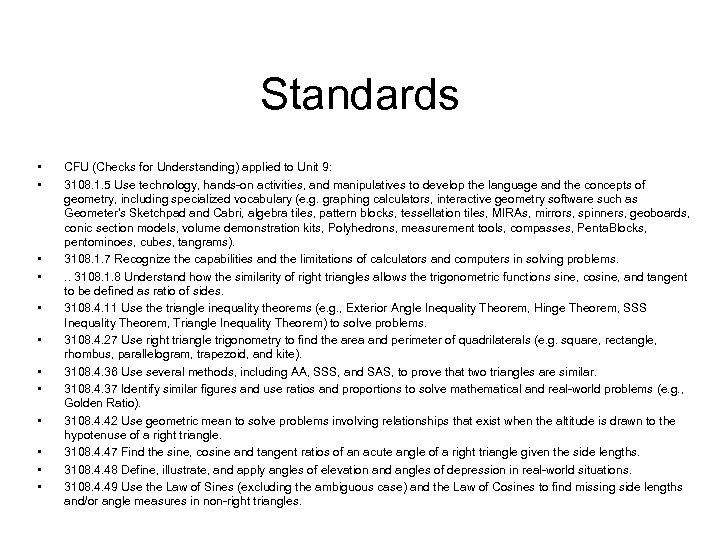Standards • • • CFU (Checks for Understanding) applied to Unit 9: 3108. 1. 5 Use technology, hands-on activities, and manipulatives to develop the language and the concepts of geometry, including specialized vocabulary (e. g. graphing calculators, interactive geometry software such as Geometer’s Sketchpad and Cabri, algebra tiles, pattern blocks, tessellation tiles, MIRAs, mirrors, spinners, geoboards, conic section models, volume demonstration kits, Polyhedrons, measurement tools, compasses, Penta. Blocks, pentominoes, cubes, tangrams). 3108. 1. 7 Recognize the capabilities and the limitations of calculators and computers in solving problems. . . 3108. 1. 8 Understand how the similarity of right triangles allows the trigonometric functions sine, cosine, and tangent to be defined as ratio of sides. 3108. 4. 11 Use the triangle inequality theorems (e. g. , Exterior Angle Inequality Theorem, Hinge Theorem, SSS Inequality Theorem, Triangle Inequality Theorem) to solve problems. 3108. 4. 27 Use right triangle trigonometry to find the area and perimeter of quadrilaterals (e. g. square, rectangle, rhombus, parallelogram, trapezoid, and kite). 3108. 4. 36 Use several methods, including AA, SSS, and SAS, to prove that two triangles are similar. 3108. 4. 37 Identify similar figures and use ratios and proportions to solve mathematical and real-world problems (e. g. , Golden Ratio). 3108. 4. 42 Use geometric mean to solve problems involving relationships that exist when the altitude is drawn to the hypotenuse of a right triangle. 3108. 4. 47 Find the sine, cosine and tangent ratios of an acute angle of a right triangle given the side lengths. 3108. 4. 48 Define, illustrate, and apply angles of elevation and angles of depression in real-world situations. 3108. 4. 49 Use the Law of Sines (excluding the ambiguous case) and the Law of Cosines to find missing side lengths and/or angle measures in non-right triangles.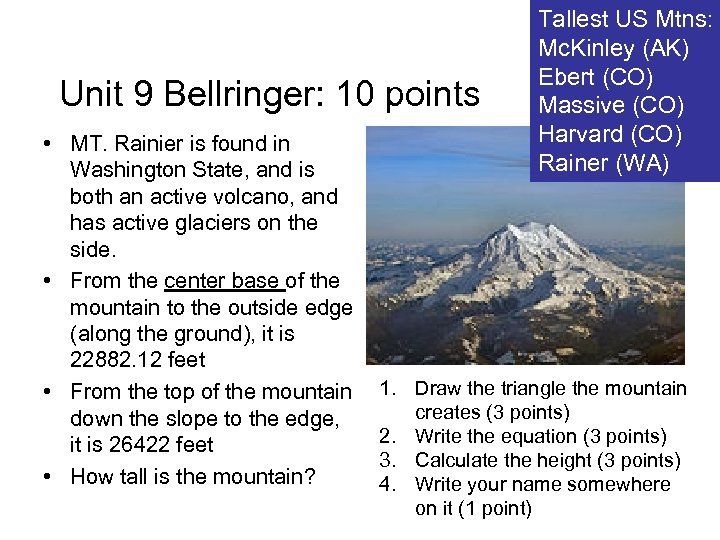Unit 9 Bellringer: 10 points • MT. Rainier is found in Washington State, and is both an active volcano, and has active glaciers on the side. • From the center base of the mountain to the outside edge (along the ground), it is 22882. 12 feet • From the top of the mountain down the slope to the edge, it is 26422 feet • How tall is the mountain? Tallest US Mtns: Mc. Kinley (AK) Ebert (CO) Massive (CO) Harvard (CO) Rainer (WA) 1. Draw the triangle the mountain creates (3 points) 2. Write the equation (3 points) 3. Calculate the height (3 points) 4. Write your name somewhere on it (1 point)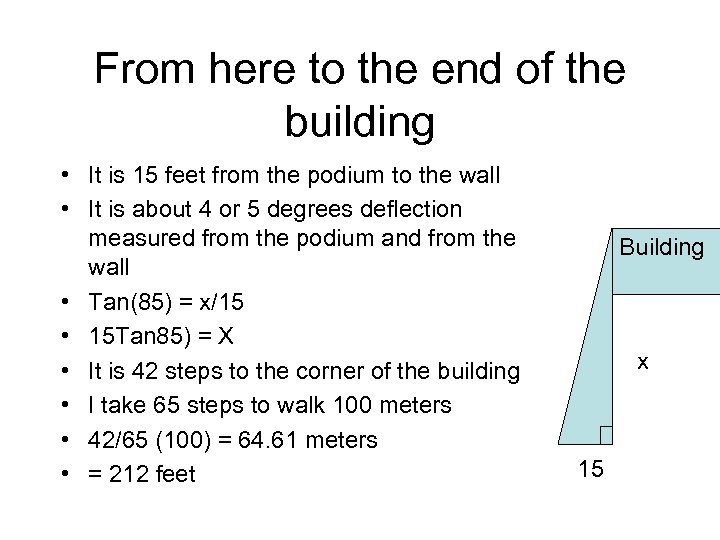From here to the end of the building • It is 15 feet from the podium to the wall • It is about 4 or 5 degrees deflection measured from the podium and from the wall • Tan(85) = x/15 • 15 Tan 85) = X • It is 42 steps to the corner of the building • I take 65 steps to walk 100 meters • 42/65 (100) = 64. 61 meters • = 212 feet Building x 15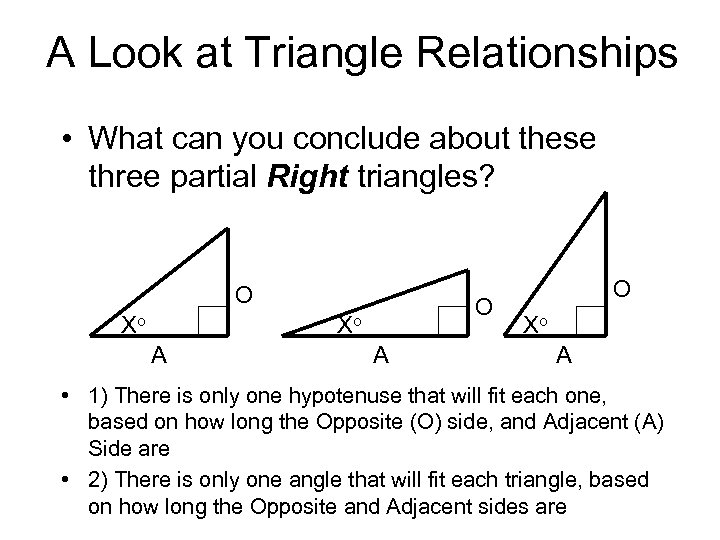A Look at Triangle Relationships • What can you conclude about these three partial Right triangles? O Xo A A O Xo A • 1) There is only one hypotenuse that will fit each one, based on how long the Opposite (O) side, and Adjacent (A) Side are • 2) There is only one angle that will fit each triangle, based on how long the Opposite and Adjacent sides are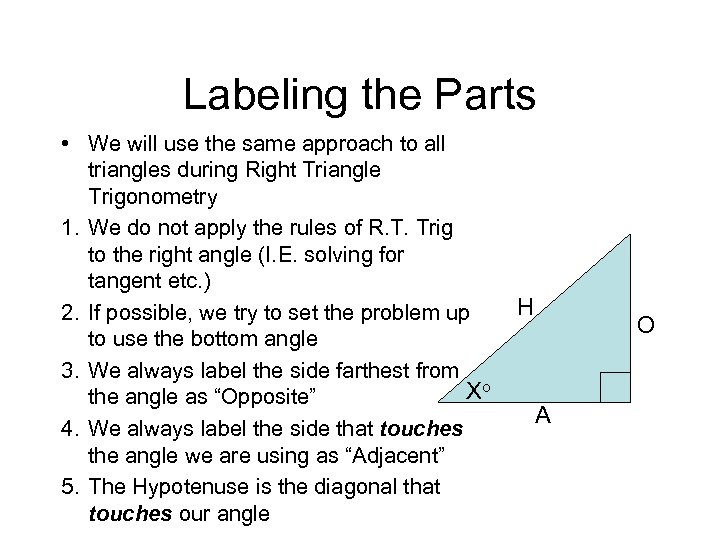Labeling the Parts • We will use the same approach to all triangles during Right Triangle Trigonometry 1. We do not apply the rules of R. T. Trig to the right angle (I. E. solving for tangent etc. ) 2. If possible, we try to set the problem up to use the bottom angle 3. We always label the side farthest from Xo the angle as “Opposite” 4. We always label the side that touches the angle we are using as “Adjacent” 5. The Hypotenuse is the diagonal that touches our angle H O A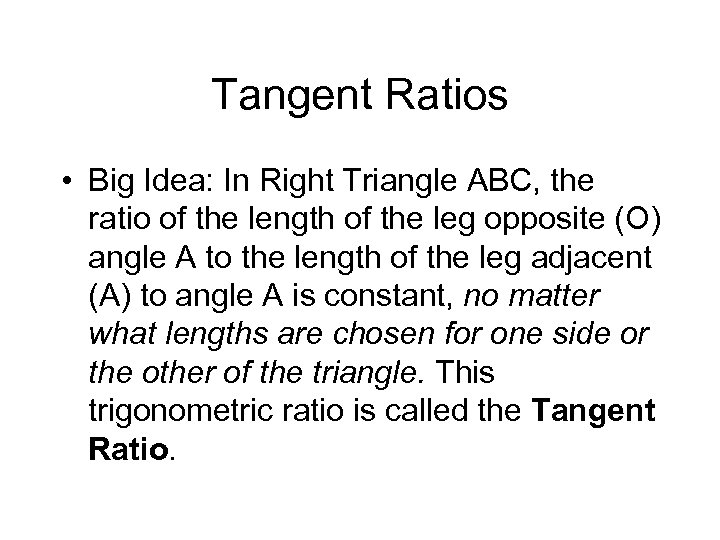Tangent Ratios • Big Idea: In Right Triangle ABC, the ratio of the length of the leg opposite (O) angle A to the length of the leg adjacent (A) to angle A is constant, no matter what lengths are chosen for one side or the other of the triangle. This trigonometric ratio is called the Tangent Ratio.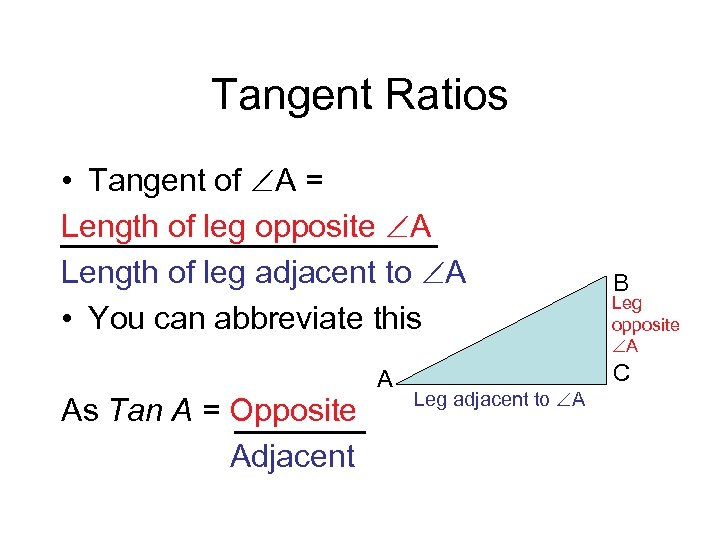Tangent Ratios • Tangent of A = Length of leg opposite A Length of leg adjacent to A • You can abbreviate this A As Tan A = Opposite Adjacent Leg adjacent to A B Leg opposite A C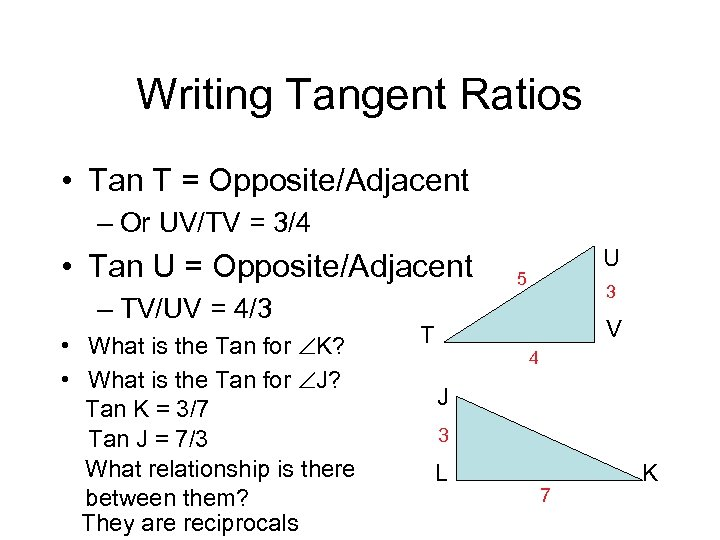Writing Tangent Ratios • Tan T = Opposite/Adjacent – Or UV/TV = 3/4 • Tan U = Opposite/Adjacent – TV/UV = 4/3 • What is the Tan for K? • What is the Tan for J? Tan K = 3/7 Tan J = 7/3 What relationship is there between them? They are reciprocals U 5 3 V T 4 J 3 L 7 K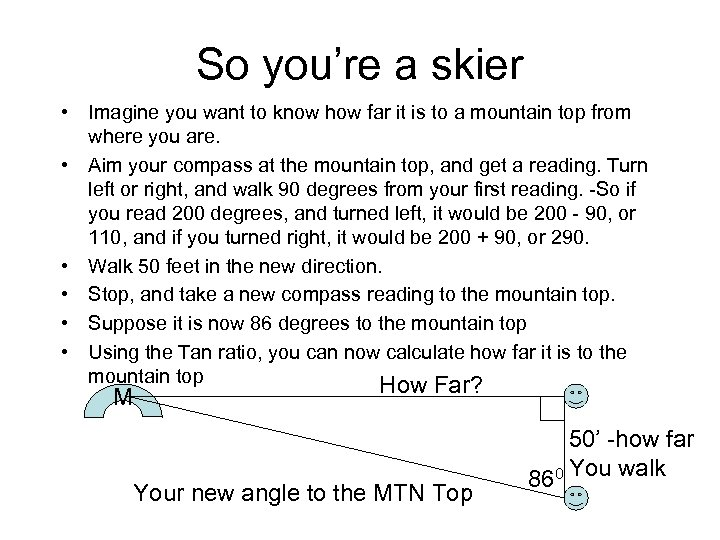So you’re a skier • Imagine you want to know how far it is to a mountain top from where you are. • Aim your compass at the mountain top, and get a reading. Turn left or right, and walk 90 degrees from your first reading. -So if you read 200 degrees, and turned left, it would be 200 - 90, or 110, and if you turned right, it would be 200 + 90, or 290. • Walk 50 feet in the new direction. • Stop, and take a new compass reading to the mountain top. • Suppose it is now 86 degrees to the mountain top • Using the Tan ratio, you can now calculate how far it is to the mountain top How Far? M Your new angle to the MTN Top 50’ -how far 860 You walk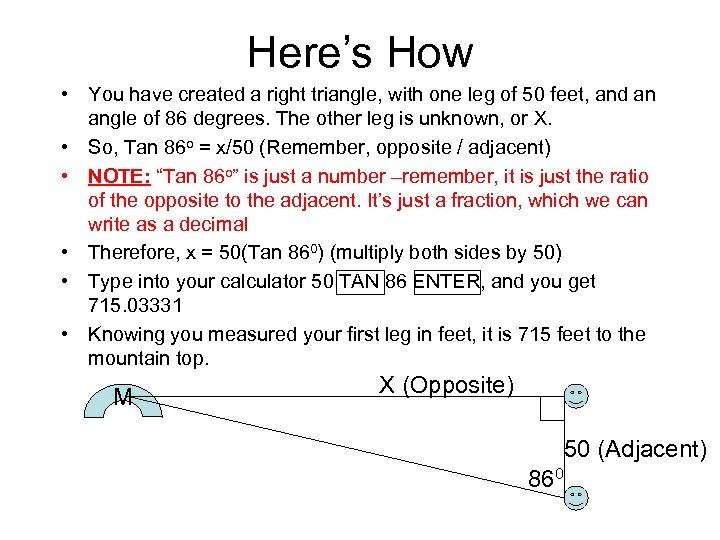Here’s How • You have created a right triangle, with one leg of 50 feet, and an angle of 86 degrees. The other leg is unknown, or X. • So, Tan 86 o = x/50 (Remember, opposite / adjacent) • NOTE: “Tan 86 o” is just a number –remember, it is just the ratio of the opposite to the adjacent. It’s just a fraction, which we can write as a decimal • Therefore, x = 50(Tan 860) (multiply both sides by 50) • Type into your calculator 50 TAN 86 ENTER, and you get 715. 03331 • Knowing you measured your first leg in feet, it is 715 feet to the mountain top. M X (Opposite) 50 (Adjacent) 860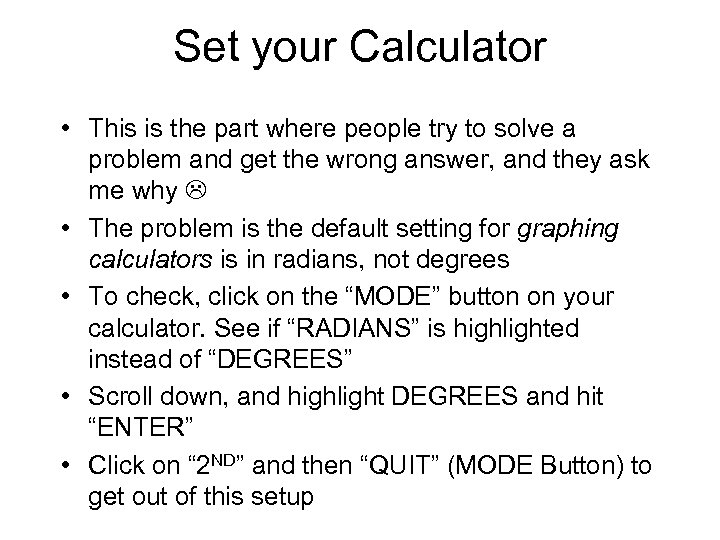Set your Calculator • This is the part where people try to solve a problem and get the wrong answer, and they ask me why • The problem is the default setting for graphing calculators is in radians, not degrees • To check, click on the “MODE” button on your calculator. See if “RADIANS” is highlighted instead of “DEGREES” • Scroll down, and highlight DEGREES and hit “ENTER” • Click on “ 2 ND” and then “QUIT” (MODE Button) to get out of this setup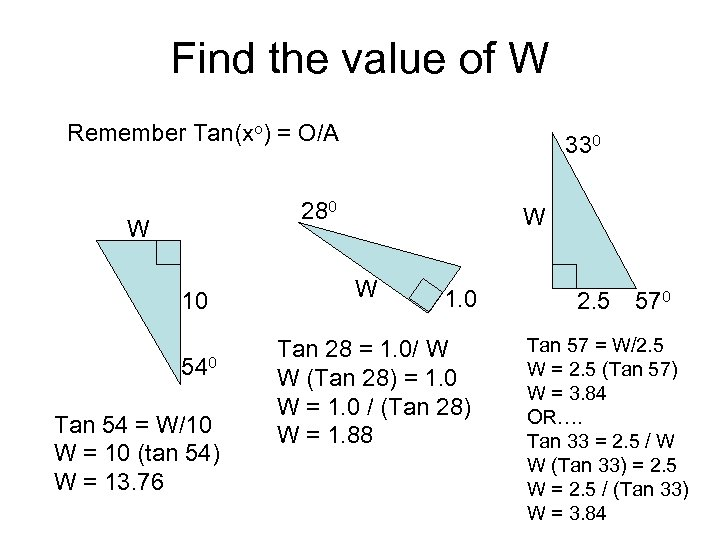Find the value of W Remember Tan(xo) = O/A 330 280 W 10 540 Tan 54 = W/10 W = 10 (tan 54) W = 13. 76 W W 1. 0 Tan 28 = 1. 0/ W W (Tan 28) = 1. 0 W = 1. 0 / (Tan 28) W = 1. 88 2. 5 570 Tan 57 = W/2. 5 W = 2. 5 (Tan 57) W = 3. 84 OR…. Tan 33 = 2. 5 / W W (Tan 33) = 2. 5 W = 2. 5 / (Tan 33) W = 3. 84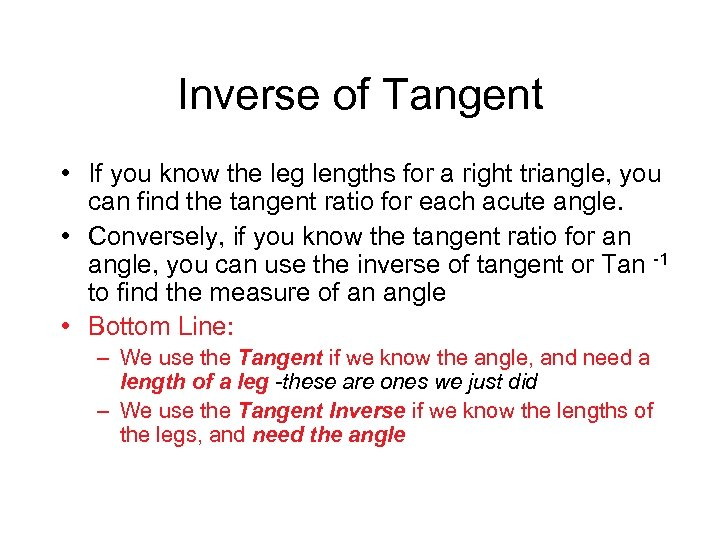Inverse of Tangent • If you know the leg lengths for a right triangle, you can find the tangent ratio for each acute angle. • Conversely, if you know the tangent ratio for an angle, you can use the inverse of tangent or Tan -1 to find the measure of an angle • Bottom Line: – We use the Tangent if we know the angle, and need a length of a leg -these are ones we just did – We use the Tangent Inverse if we know the lengths of the legs, and need the angle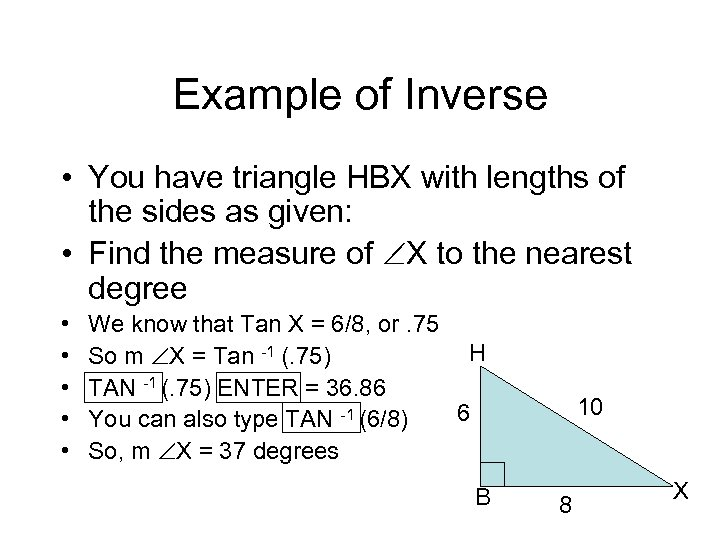Example of Inverse • You have triangle HBX with lengths of the sides as given: • Find the measure of X to the nearest degree • • • We know that Tan X = 6/8, or. 75 H So m X = Tan -1 (. 75) TAN -1 (. 75) ENTER = 36. 86 6 You can also type TAN -1 (6/8) So, m X = 37 degrees B 10 8 X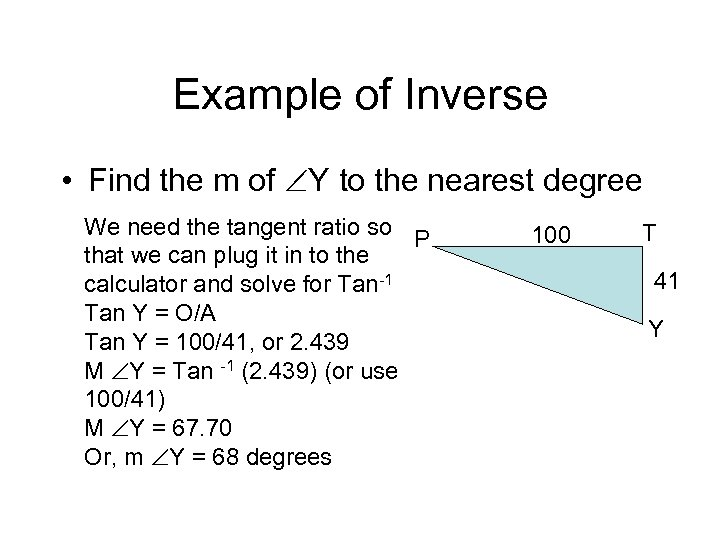Example of Inverse • Find the m of Y to the nearest degree We need the tangent ratio so P that we can plug it in to the calculator and solve for Tan-1 Tan Y = O/A Tan Y = 100/41, or 2. 439 M Y = Tan -1 (2. 439) (or use 100/41) M Y = 67. 70 Or, m Y = 68 degrees 100 T 41 Y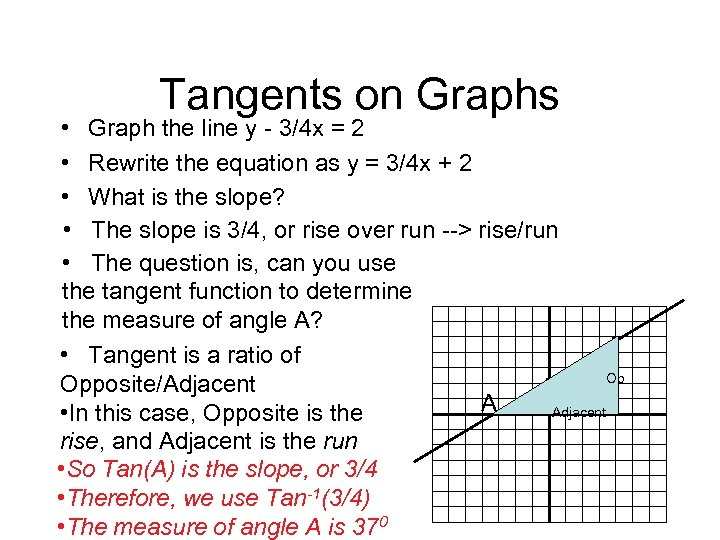Tangents on Graphs • Graph the line y - 3/4 x = 2 • Rewrite the equation as y = 3/4 x + 2 • What is the slope? • The slope is 3/4, or rise over run --> rise/run • The question is, can you use the tangent function to determine the measure of angle A? • Tangent is a ratio of Op Opposite/Adjacent A Adjacent • In this case, Opposite is the rise, and Adjacent is the run • So Tan(A) is the slope, or 3/4 • Therefore, we use Tan-1(3/4) • The measure of angle A is 370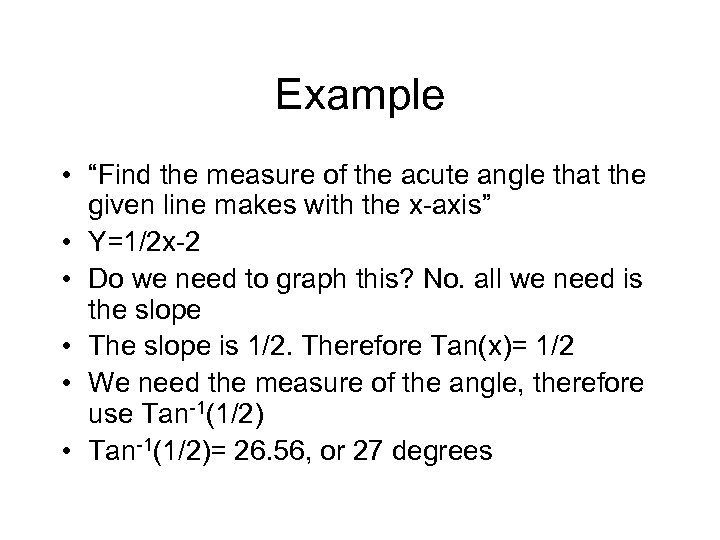Example • “Find the measure of the acute angle that the given line makes with the x-axis” • Y=1/2 x-2 • Do we need to graph this? No. all we need is the slope • The slope is 1/2. Therefore Tan(x)= 1/2 • We need the measure of the angle, therefore use Tan-1(1/2) • Tan-1(1/2)= 26. 56, or 27 degrees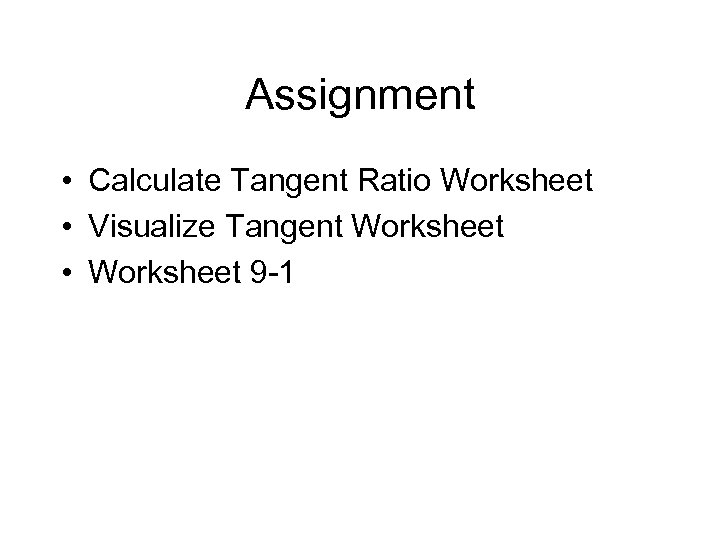Assignment • Calculate Tangent Ratio Worksheet • Visualize Tangent Worksheet • Worksheet 9 -1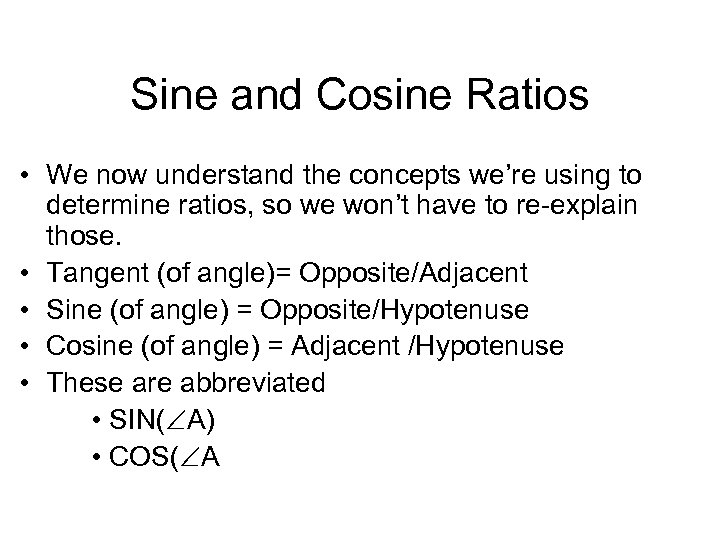Sine and Cosine Ratios • We now understand the concepts we’re using to determine ratios, so we won’t have to re-explain those. • Tangent (of angle)= Opposite/Adjacent • Sine (of angle) = Opposite/Hypotenuse • Cosine (of angle) = Adjacent /Hypotenuse • These are abbreviated • SIN( A) • COS( A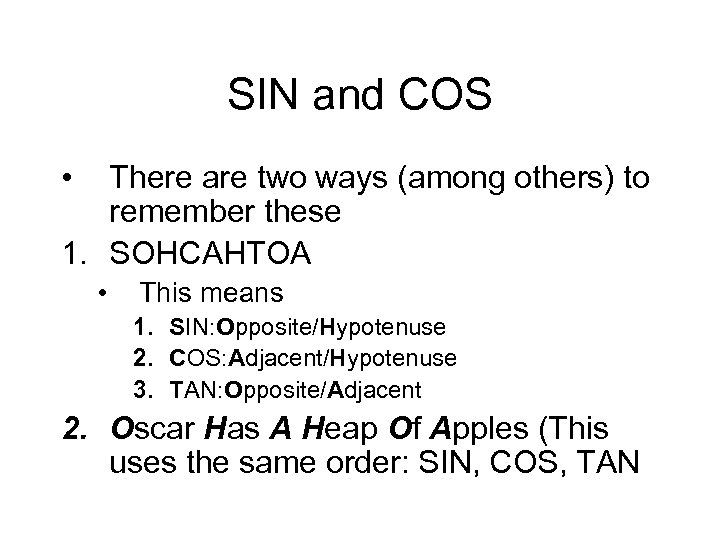SIN and COS • There are two ways (among others) to remember these 1. SOHCAHTOA • This means 1. SIN: Opposite/Hypotenuse 2. COS: Adjacent/Hypotenuse 3. TAN: Opposite/Adjacent 2. Oscar Has A Heap Of Apples (This uses the same order: SIN, COS, TAN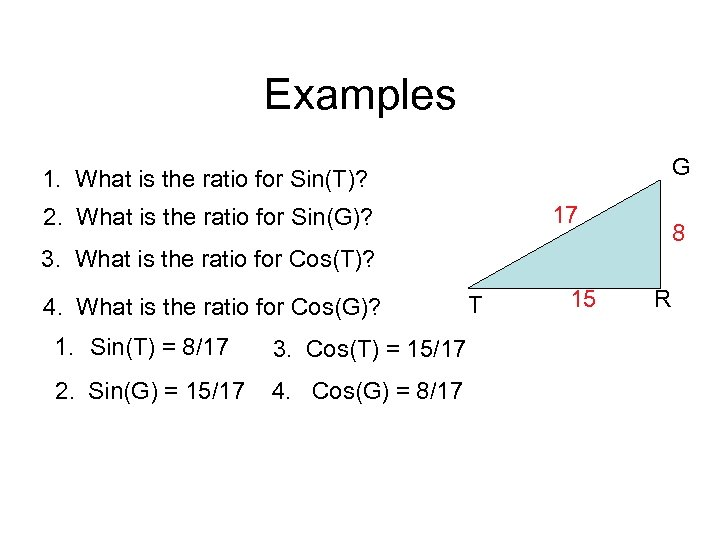Examples G 1. What is the ratio for Sin(T)? 17 2. What is the ratio for Sin(G)? 8 3. What is the ratio for Cos(T)? 4. What is the ratio for Cos(G)? 1. Sin(T) = 8/17 3. Cos(T) = 15/17 2. Sin(G) = 15/17 4. Cos(G) = 8/17 T 15 R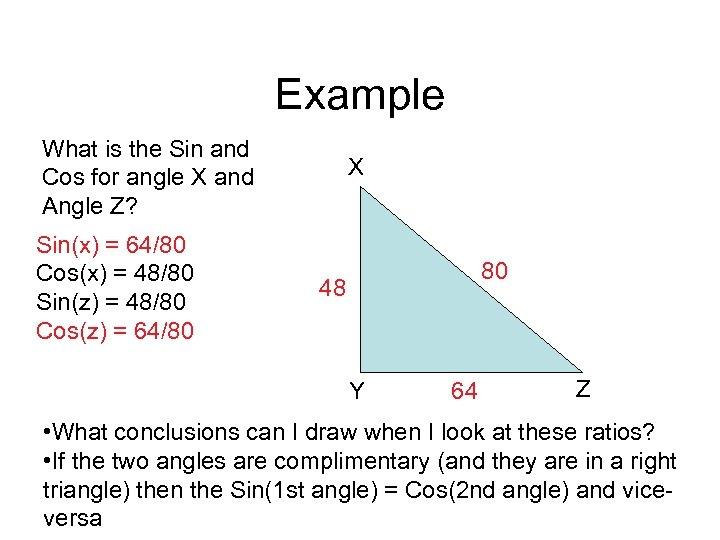Example What is the Sin and Cos for angle X and Angle Z? Sin(x) = 64/80 Cos(x) = 48/80 Sin(z) = 48/80 Cos(z) = 64/80 X 80 48 Y 64 Z • What conclusions can I draw when I look at these ratios? • If the two angles are complimentary (and they are in a right triangle) then the Sin(1 st angle) = Cos(2 nd angle) and viceversa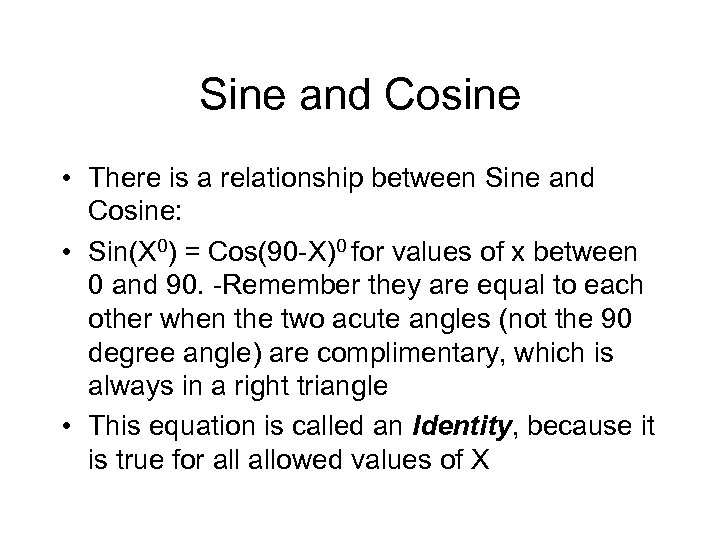Sine and Cosine • There is a relationship between Sine and Cosine: • Sin(X 0) = Cos(90 -X)0 for values of x between 0 and 90. -Remember they are equal to each other when the two acute angles (not the 90 degree angle) are complimentary, which is always in a right triangle • This equation is called an Identity, because it is true for allowed values of X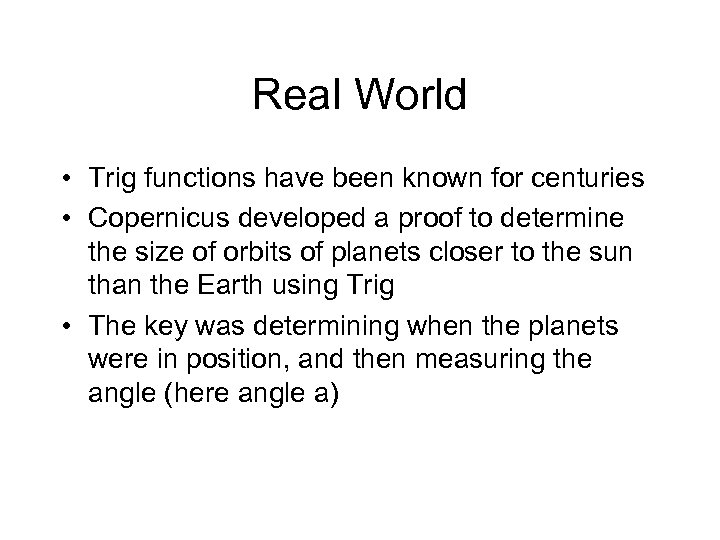Real World • Trig functions have been known for centuries • Copernicus developed a proof to determine the size of orbits of planets closer to the sun than the Earth using Trig • The key was determining when the planets were in position, and then measuring the angle (here angle a)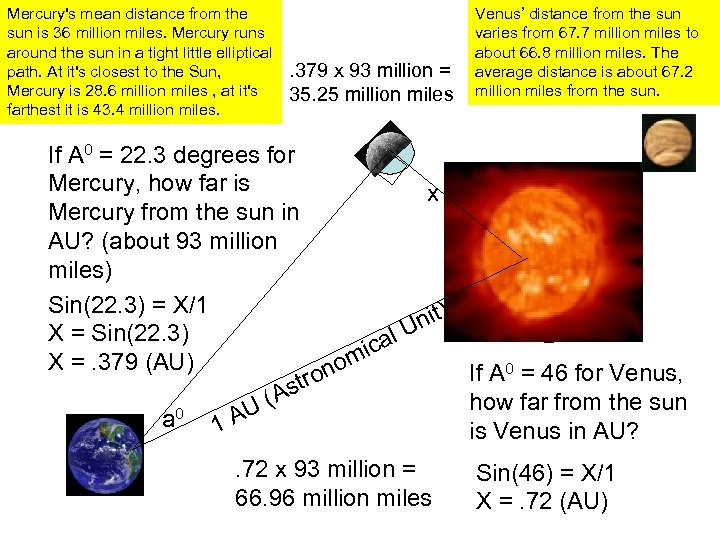Mercury's mean distance from the sun is 36 million miles. Mercury runs around the sun in a tight little elliptical path. At it's closest to the Sun, Mercury is 28. 6 million miles , at it's farthest it is 43. 4 million miles. . 379 x 93 million = 35. 25 million miles Real World Venus’ distance from the sun varies from 67. 7 million miles to about 66. 8 million miles. The average distance is about 67. 2 million miles from the sun. If A 0 = 22. 3 degrees for Mercury, how far is x Mercury from the sun in AU? (about 93 million Sun miles) Sin(22. 3) = X/1 nit) X = Sin(22. 3) al U mic X =. 379 (AU) o If A 0 = 46 for Venus, on r t As how far from the sun U( 0 a 1 A is Venus in AU? . 72 x 93 million = 66. 96 million miles Sin(46) = X/1 X =. 72 (AU)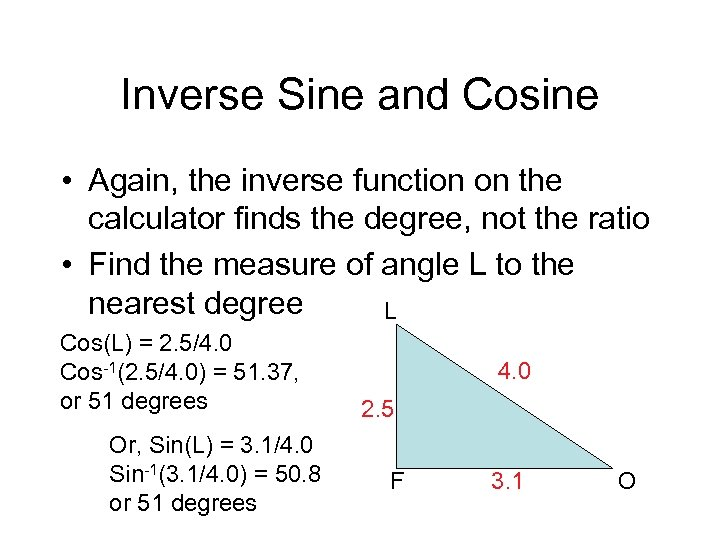Inverse Sine and Cosine • Again, the inverse function on the calculator finds the degree, not the ratio • Find the measure of angle L to the nearest degree L Cos(L) = 2. 5/4. 0 Cos-1(2. 5/4. 0) = 51. 37, or 51 degrees Or, Sin(L) = 3. 1/4. 0 Sin-1(3. 1/4. 0) = 50. 8 or 51 degrees 4. 0 2. 5 F 3. 1 O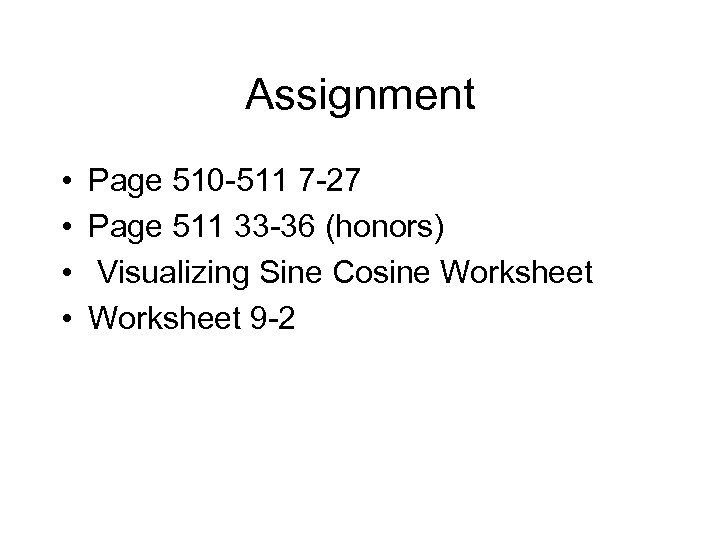Assignment • • Page 510 -511 7 -27 Page 511 33 -36 (honors) Visualizing Sine Cosine Worksheet 9 -2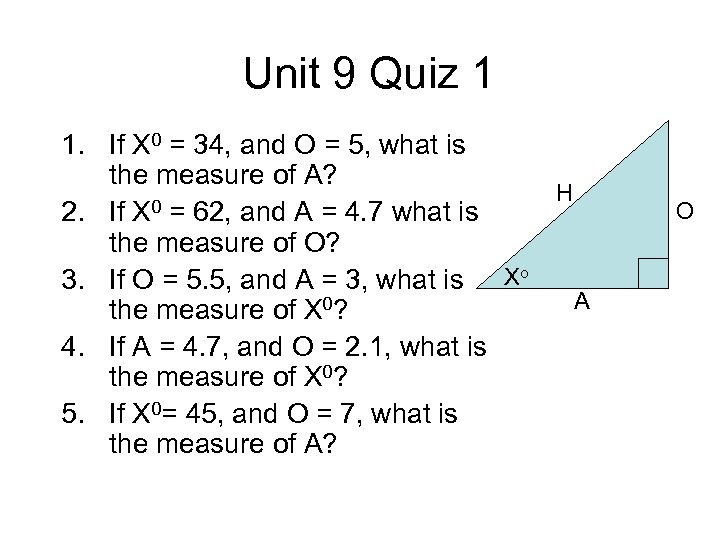Unit 9 Quiz 1 1. If X 0 = 34, and O = 5, what is the measure of A? H 0 = 62, and A = 4. 7 what is 2. If X the measure of O? 3. If O = 5. 5, and A = 3, what is Xo A the measure of X 0? 4. If A = 4. 7, and O = 2. 1, what is the measure of X 0? 5. If X 0= 45, and O = 7, what is the measure of A? O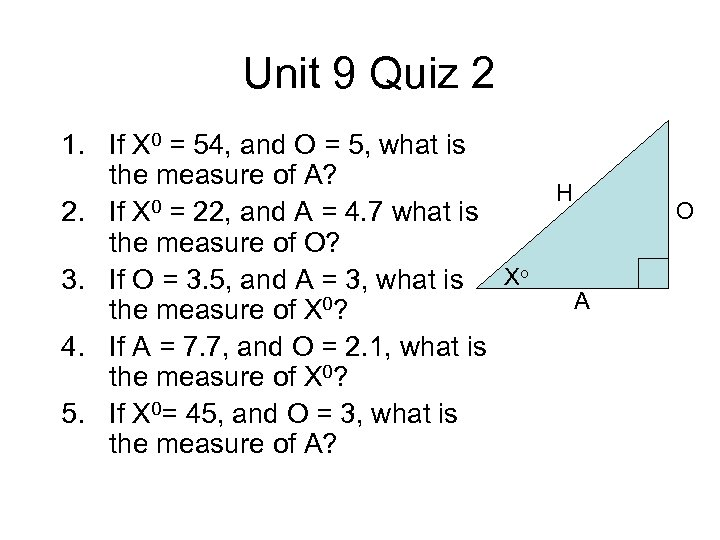Unit 9 Quiz 2 1. If X 0 = 54, and O = 5, what is the measure of A? H 0 = 22, and A = 4. 7 what is 2. If X the measure of O? 3. If O = 3. 5, and A = 3, what is Xo A the measure of X 0? 4. If A = 7. 7, and O = 2. 1, what is the measure of X 0? 5. If X 0= 45, and O = 3, what is the measure of A? O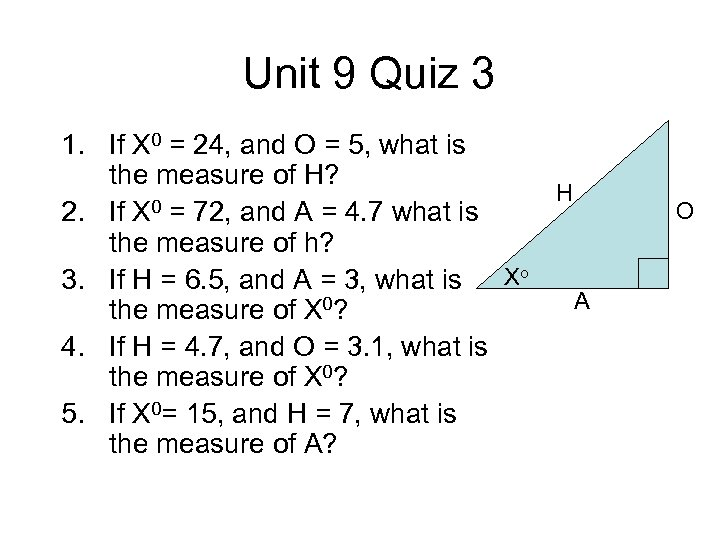Unit 9 Quiz 3 1. If X 0 = 24, and O = 5, what is the measure of H? H 0 = 72, and A = 4. 7 what is 2. If X the measure of h? 3. If H = 6. 5, and A = 3, what is Xo A the measure of X 0? 4. If H = 4. 7, and O = 3. 1, what is the measure of X 0? 5. If X 0= 15, and H = 7, what is the measure of A? O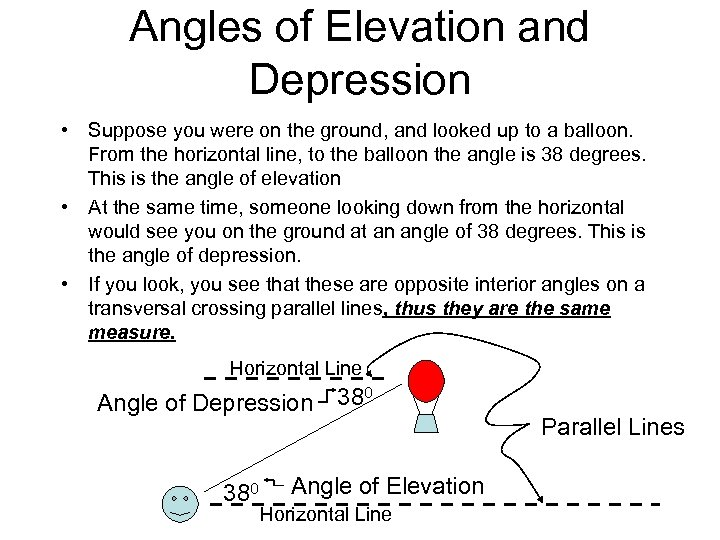Angles of Elevation and Depression • Suppose you were on the ground, and looked up to a balloon. From the horizontal line, to the balloon the angle is 38 degrees. This is the angle of elevation • At the same time, someone looking down from the horizontal would see you on the ground at an angle of 38 degrees. This is the angle of depression. • If you look, you see that these are opposite interior angles on a transversal crossing parallel lines, thus they are the same measure. Horizontal Line Angle of Depression 380 Angle of Elevation Horizontal Line Parallel Lines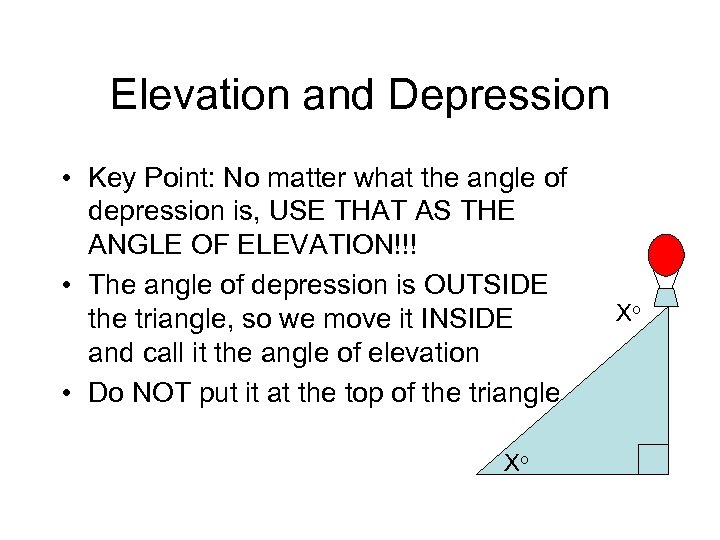Elevation and Depression • Key Point: No matter what the angle of depression is, USE THAT AS THE ANGLE OF ELEVATION!!! • The angle of depression is OUTSIDE the triangle, so we move it INSIDE and call it the angle of elevation • Do NOT put it at the top of the triangle Xo Xo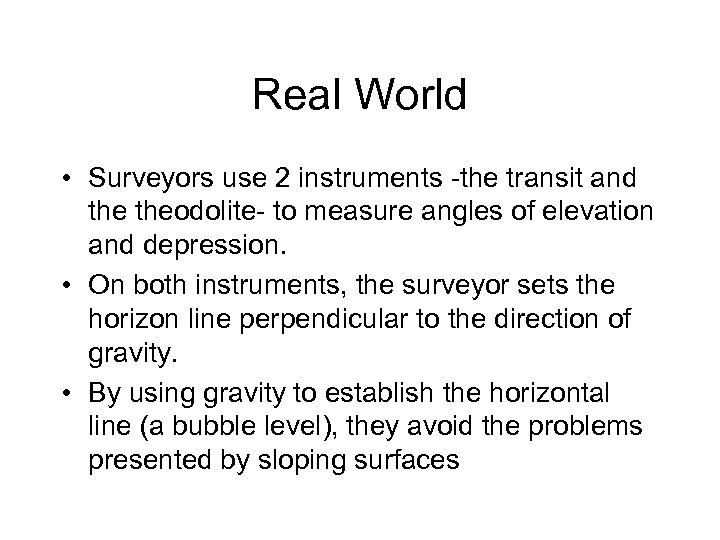Real World • Surveyors use 2 instruments -the transit and theodolite- to measure angles of elevation and depression. • On both instruments, the surveyor sets the horizon line perpendicular to the direction of gravity. • By using gravity to establish the horizontal line (a bubble level), they avoid the problems presented by sloping surfacesReal World • A surveyor wants to find the height of the “Delicate Arch” in Arches National Park in Utah. • To do this, she sets theodolite at the bottom of the arch, and moves to a point where she can measure the angle to the top • Then she measures how far she walked out to measure the arch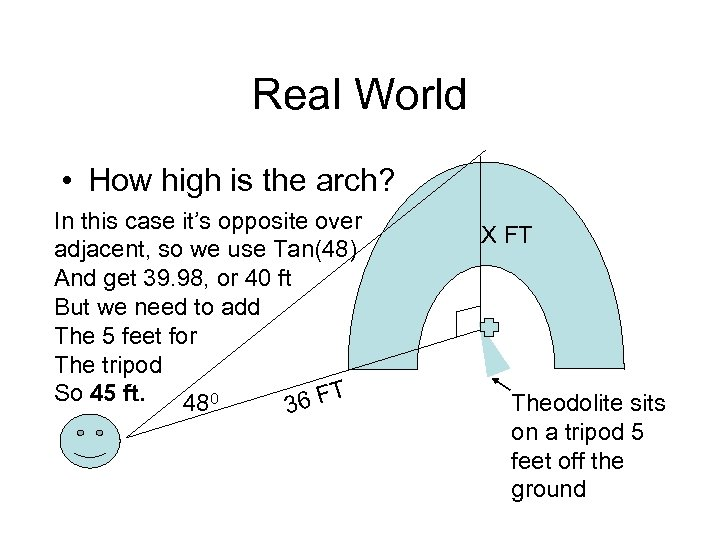Real World • How high is the arch? In this case it’s opposite over adjacent, so we use Tan(48) And get 39. 98, or 40 ft But we need to add The 5 feet for The tripod So 45 ft. 6 FT 480 3 X FT Theodolite sits on a tripod 5 feet off the ground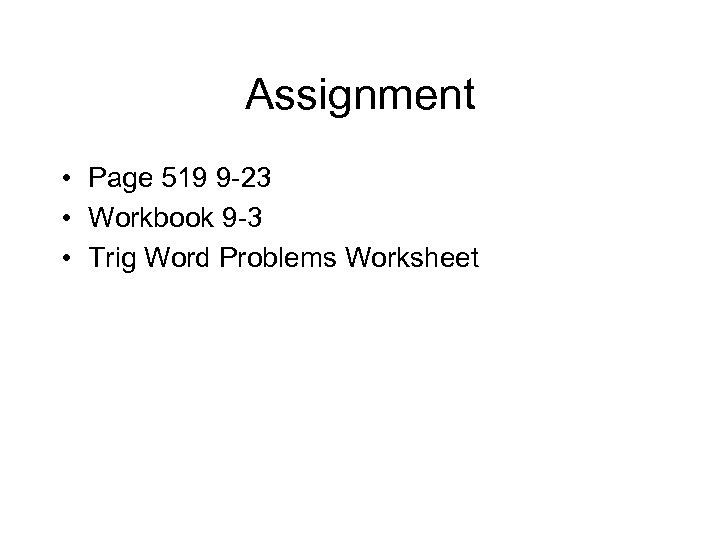Assignment • Page 519 9 -23 • Workbook 9 -3 • Trig Word Problems Worksheet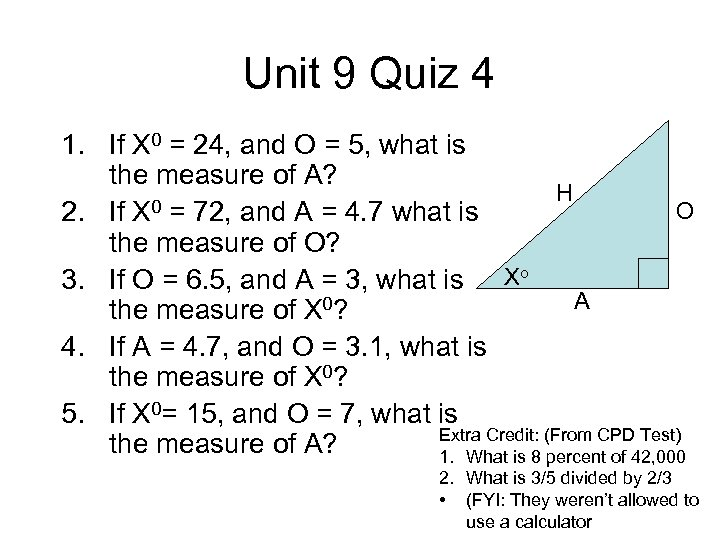Unit 9 Quiz 4 1. If X 0 = 24, and O = 5, what is the measure of A? H 0 = 72, and A = 4. 7 what is O 2. If X the measure of O? 3. If O = 6. 5, and A = 3, what is Xo A the measure of X 0? 4. If A = 4. 7, and O = 3. 1, what is the measure of X 0? 5. If X 0= 15, and O = 7, what is Extra Credit: (From CPD Test) the measure of A? 1. What is 8 percent of 42, 000 2. What is 3/5 divided by 2/3 • (FYI: They weren’t allowed to use a calculator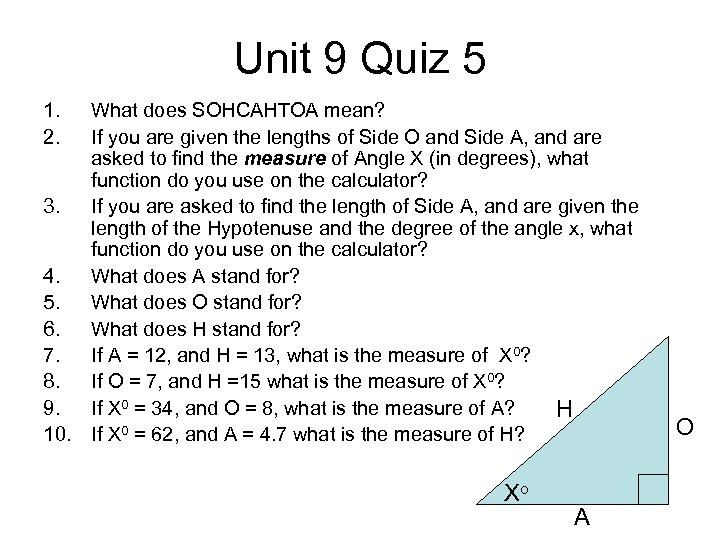Unit 9 Quiz 5 1. 2. What does SOHCAHTOA mean? If you are given the lengths of Side O and Side A, and are asked to find the measure of Angle X (in degrees), what function do you use on the calculator? 3. If you are asked to find the length of Side A, and are given the length of the Hypotenuse and the degree of the angle x, what function do you use on the calculator? 4. What does A stand for? 5. What does O stand for? 6. What does H stand for? 7. If A = 12, and H = 13, what is the measure of X 0? 8. If O = 7, and H =15 what is the measure of X 0? 9. If X 0 = 34, and O = 8, what is the measure of A? H 10. If X 0 = 62, and A = 4. 7 what is the measure of H? Xo A O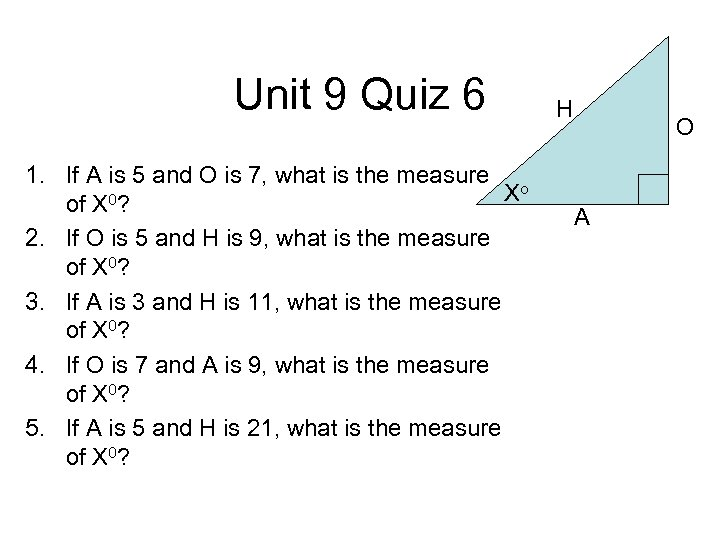Unit 9 Quiz 6 1. If A is 5 and O is 7, what is the measure o X of X 0? 2. If O is 5 and H is 9, what is the measure of X 0? 3. If A is 3 and H is 11, what is the measure of X 0? 4. If O is 7 and A is 9, what is the measure of X 0? 5. If A is 5 and H is 21, what is the measure of X 0? H O A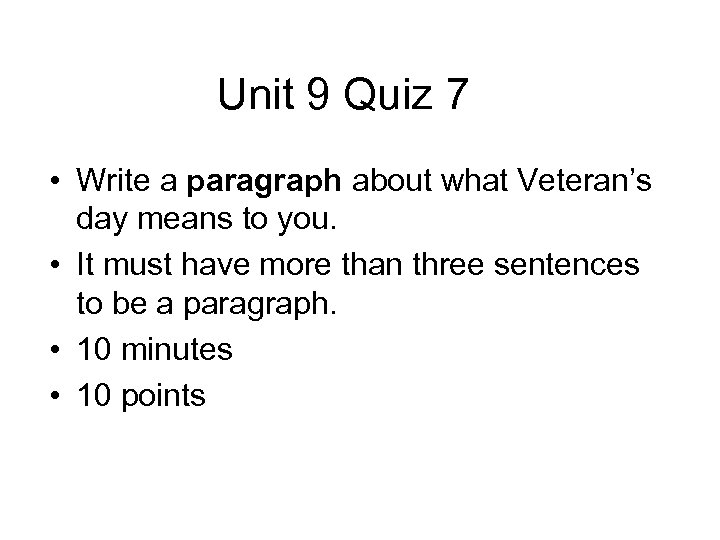Unit 9 Quiz 7 • Write a paragraph about what Veteran’s day means to you. • It must have more than three sentences to be a paragraph. • 10 minutes • 10 points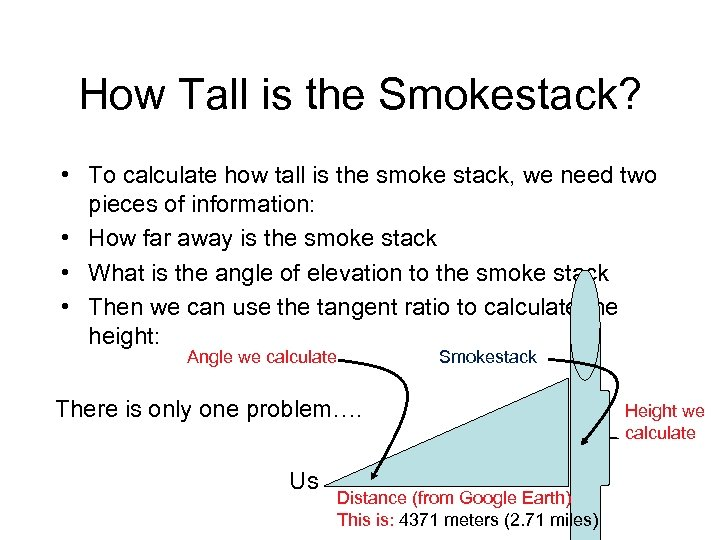How Tall is the Smokestack? • To calculate how tall is the smoke stack, we need two pieces of information: • How far away is the smoke stack • What is the angle of elevation to the smoke stack • Then we can use the tangent ratio to calculate the height: Angle we calculate Smokestack There is only one problem…. Us Distance (from Google Earth) This is: 4371 meters (2. 71 miles) Height we calculate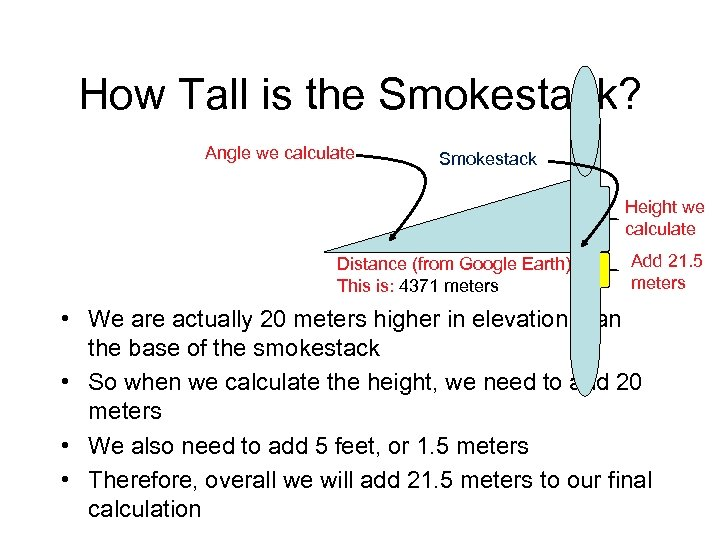How Tall is the Smokestack? Angle we calculate Smokestack Height we calculate Distance (from Google Earth) This is: 4371 meters Add 21. 5 meters • We are actually 20 meters higher in elevation than the base of the smokestack • So when we calculate the height, we need to add 20 meters • We also need to add 5 feet, or 1. 5 meters • Therefore, overall we will add 21. 5 meters to our final calculation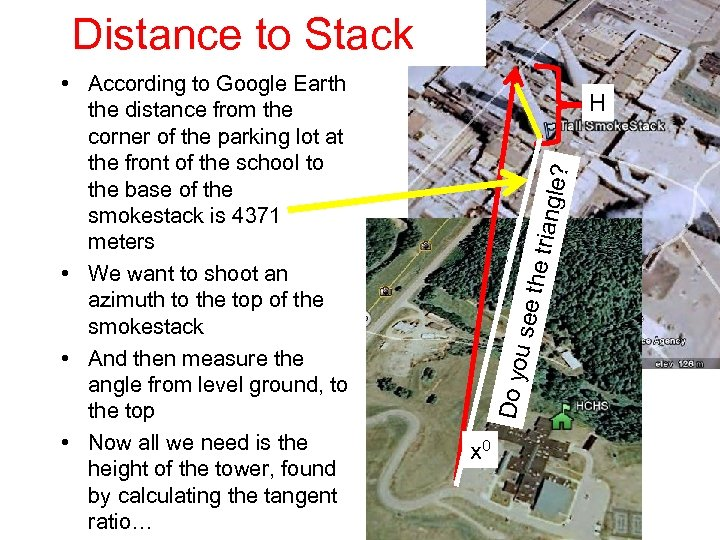Distance to Stack u see the tri angle ? H Do yo • According to Google Earth the distance from the corner of the parking lot at the front of the school to the base of the smokestack is 4371 meters • We want to shoot an azimuth to the top of the smokestack • And then measure the angle from level ground, to the top • Now all we need is the height of the tower, found by calculating the tangent ratio… x 0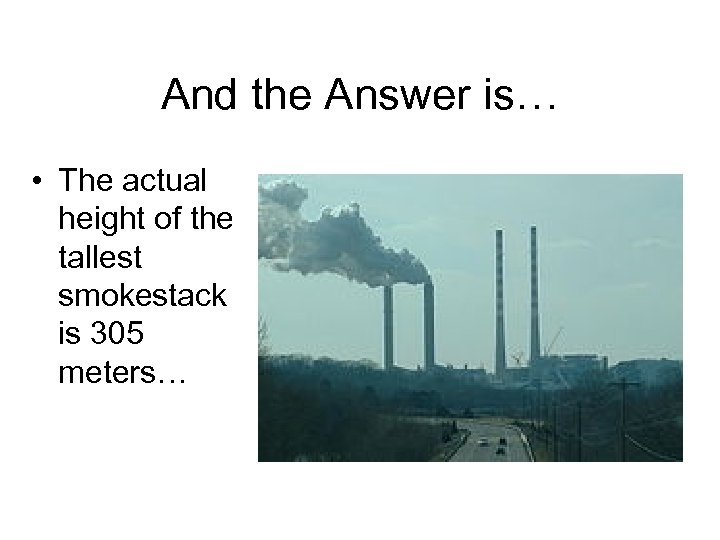And the Answer is… • The actual height of the tallest smokestack is 305 meters…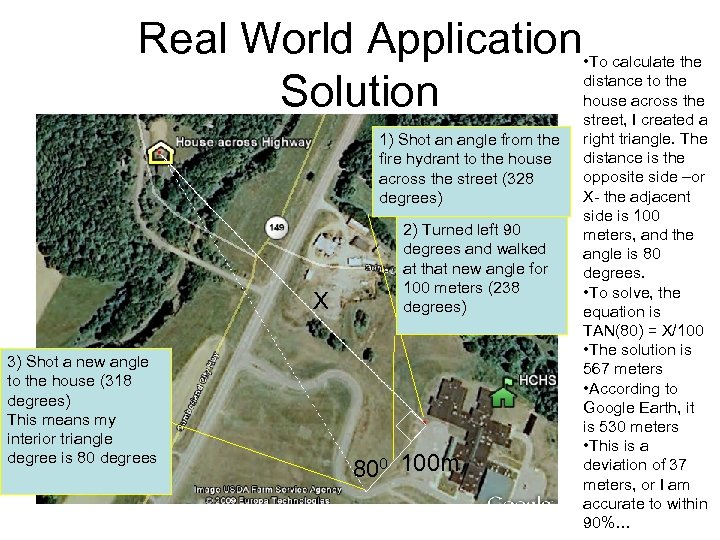Real World Application Solution 1) Shot an angle from the fire hydrant to the house across the street (328 degrees) X 3) Shot a new angle to the house (318 degrees) This means my interior triangle degree is 80 degrees 2) Turned left 90 degrees and walked at that new angle for 100 meters (238 degrees) 800 100 m • To calculate the distance to the house across the street, I created a right triangle. The distance is the opposite side –or X- the adjacent side is 100 meters, and the angle is 80 degrees. • To solve, the equation is TAN(80) = X/100 • The solution is 567 meters • According to Google Earth, it is 530 meters • This is a deviation of 37 meters, or I am accurate to within 90%…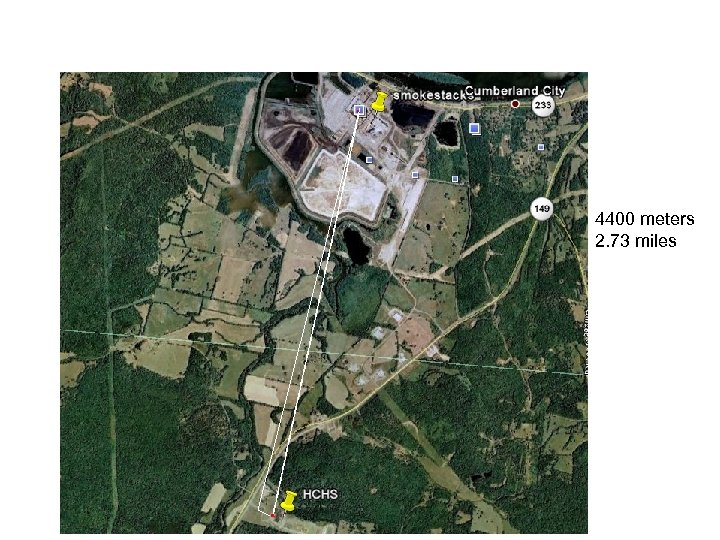4400 meters 2. 73 miles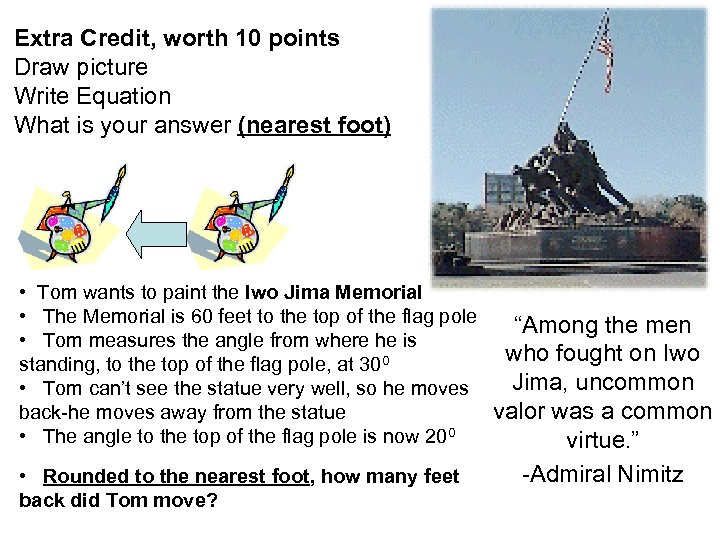Extra Credit, worth 10 points Draw picture Write Equation What is your answer (nearest foot) • Tom wants to paint the Iwo Jima Memorial • The Memorial is 60 feet to the top of the flag pole “Among the men • Tom measures the angle from where he is who fought on Iwo standing, to the top of the flag pole, at 300 Jima, uncommon • Tom can’t see the statue very well, so he moves back-he moves away from the statue valor was a common • The angle to the top of the flag pole is now 200 virtue. ” • Rounded to the nearest foot, how many feet back did Tom move? -Admiral Nimitz